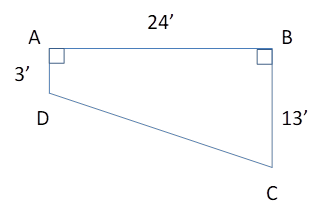Chapter 4.4, Problem 40E### Elementary Geometry for College St...

6th Edition
Daniel C. Alexander + 1 other
ISBN: 9781285195698

#### Solutions

Chapter
Section### Elementary Geometry for College St...

6th Edition
Daniel C. Alexander + 1 other
ISBN: 9781285195698
Textbook Problem
1 views

# For the in-ground pool shown in Exercise 41, find the length of the sloped bottom from point D to point C.To determine

To Find:

The length of the sloped bottom.

Explanation

Consider the following diagram.

Consider the triangle ΔABD.

Now, (BD)2=(24)2+(3)2=576+9=585.

Therefore, BD=24

### Still sussing out bartleby?

Check out a sample textbook solution.

See a sample solution

#### The Solution to Your Study Problems

Bartleby provides explanations to thousands of textbook problems written by our experts, many with advanced degrees!

Get Started